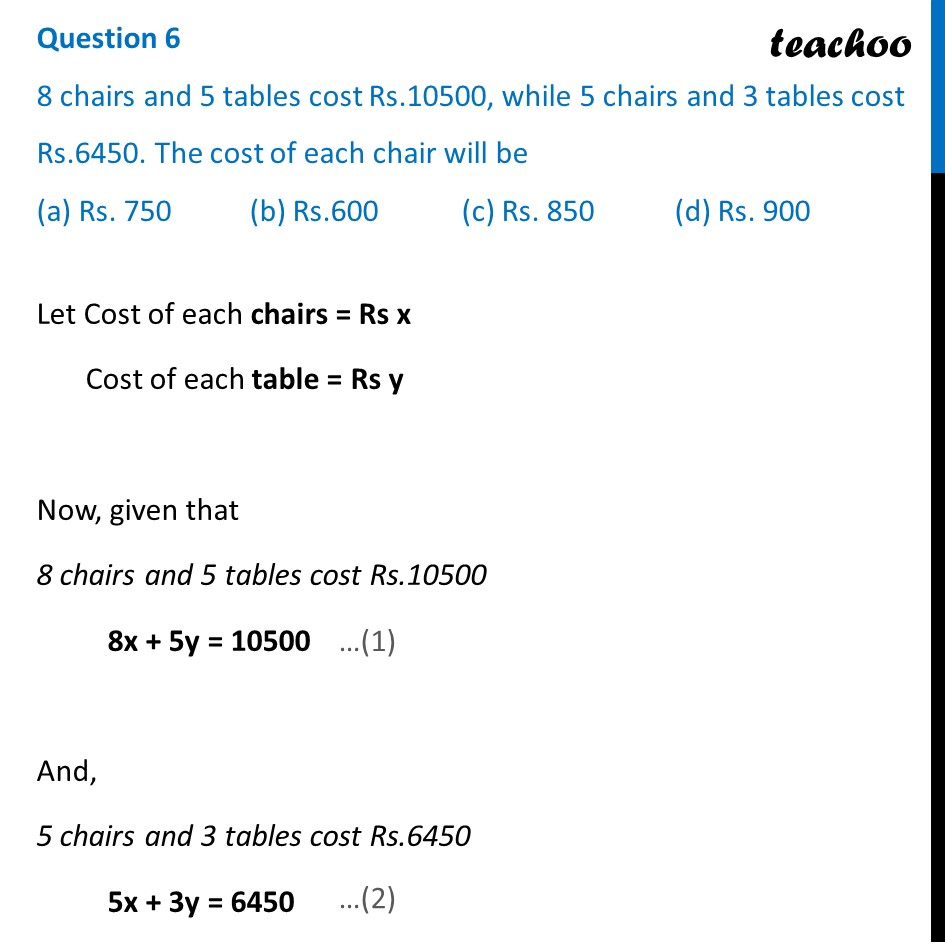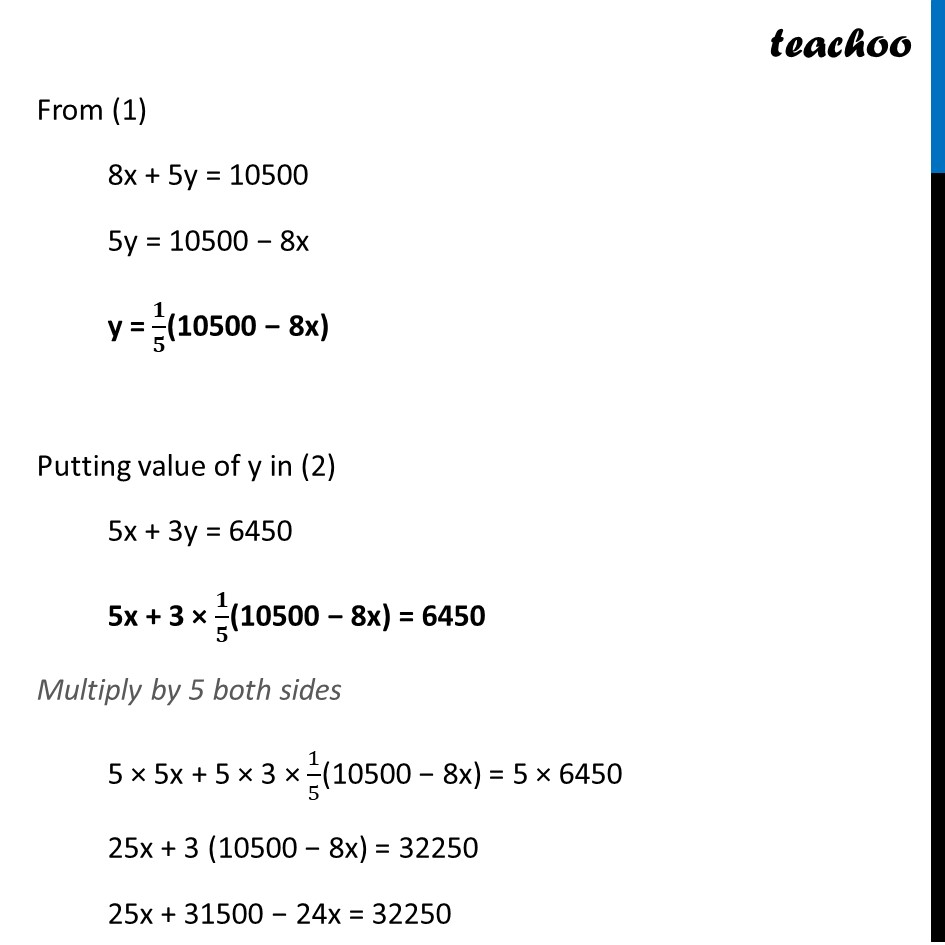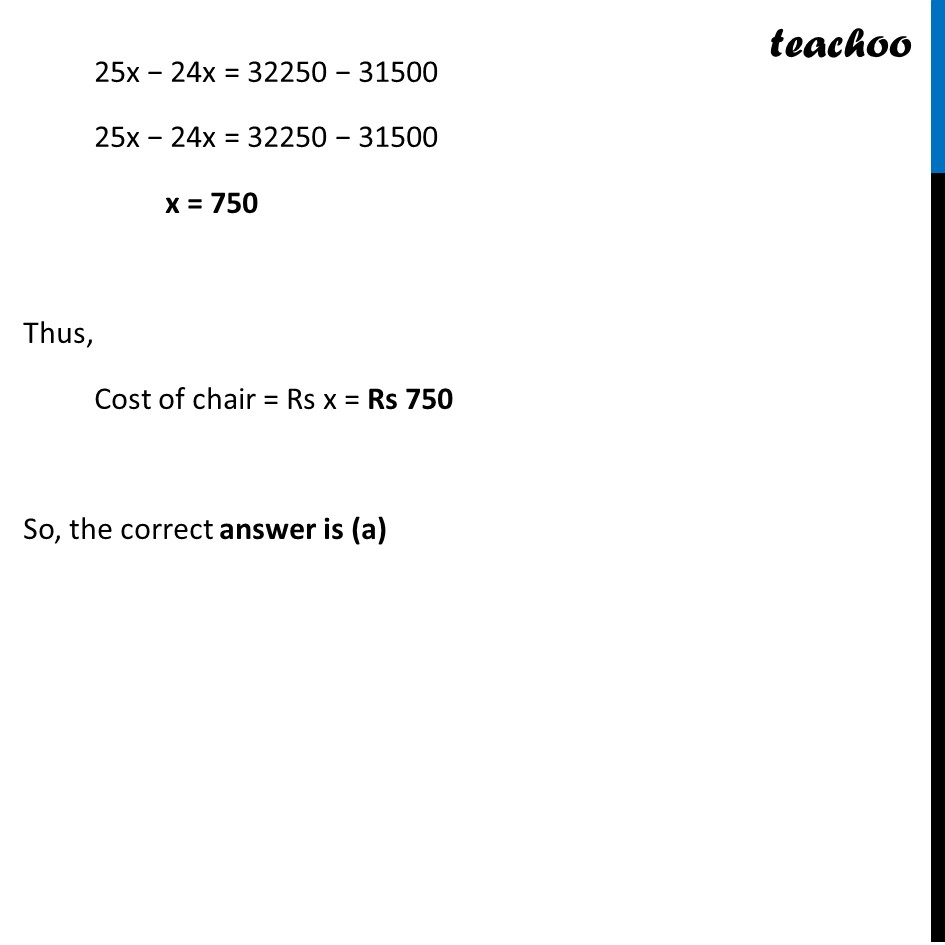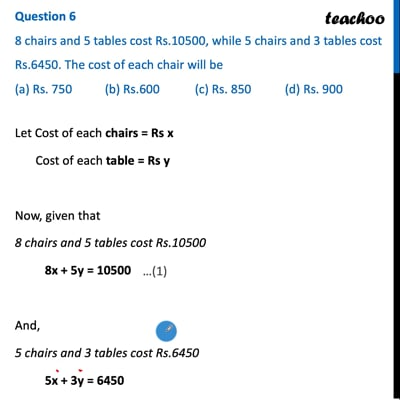CBSE Class 10 Sample Paper for 2022 Boards - Maths Basic [MCQ]

Class 10
Solutions of Sample Papers for Class 10 Boards

## (a) Rs. 750   (b) Rs.600   (c) Rs. 850   (d) Rs. 900This video is only available for Teachoo black users

Introducing your new favourite teacher - Teachoo Black, at only ₹83 per month

### Transcript

Question 6 8 chairs and 5 tables cost Rs.10500, while 5 chairs and 3 tables cost Rs.6450. The cost of each chair will be (a) Rs. 750 (b) Rs.600 (c) Rs. 850 (d) Rs. 900 Let Cost of each chairs = Rs x Cost of each table = Rs y Now, given that 8 chairs and 5 tables cost Rs.10500 8x + 5y = 10500 And, 5 chairs and 3 tables cost Rs.6450 5x + 3y = 6450 Let Cost of each chairs = Rs x Cost of each table = Rs y Now, given that 8 chairs and 5 tables cost Rs.10500 8x + 5y = 10500 And, 5 chairs and 3 tables cost Rs.6450 5x + 3y = 6450 From (1) 8x + 5y = 10500 5y = 10500 − 8x y = 𝟏/𝟓(10500 − 8x) Putting value of y in (2) 5x + 3y = 6450 5x + 3 × 𝟏/𝟓(10500 − 8x) = 6450 Multiply by 5 both sides 5 × 5x + 5 × 3 × 1/5(10500 − 8x) = 5 × 6450 25x + 3 (10500 − 8x) = 32250 25x + 31500 − 24x = 32250 25x − 24x = 32250 − 31500 25x − 24x = 32250 − 31500 x = 750 Thus, Cost of chair = Rs x = Rs 750 So, the correct answer is (a)